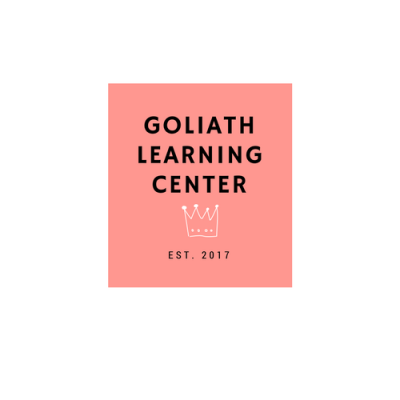Goliath Learning Center is an educational entity that offers an individualized learning experience.

## Kindergarten

• Number Counting
• Whole Numbers
• Number Comparison
• Geometric Shapes
• Data Analysis
• Financial Literacy
• Whole Numbers
• Number Comparison
• Currency
• Number Patterns
• Geometric Shapes
• Data Analysis
• Financial Literacy
• Whole Numbers
• Number Comparison
• Fractions
• Multiplication/Division (Equal Groupings)
• Monetary Transactions
• Number Patterns
• Geometric Shapes
• Measurement (Length, Area, Time)
• Data Analysis- Graphs
• Financial Literacy

• Whole Numbers
• Place Value
• Fractions
• Rounding
• Multiplication/Division
• Area/Volume
• Data Analysis- Graphs
• Financial Literacy
• Math Facts
• Whole Numbers
•  Decimals
• Place Value
• Fractions
• Perimeter/Area
• Angles
• Volume, Time, Length
• Data Analysis
• Financial Literacy
• Math Facts
• Whole Numbers
• Rational Numbers
• Algebraic Expressions
• Measurement
• Volume
• Measurement System- Customary/Metric
• Coordinate Plane
• Financial Literacy
• Math Facts